# Chemistry 100 Chapter 7 Chemical Reactions Chemical Reactions

• Slides: 49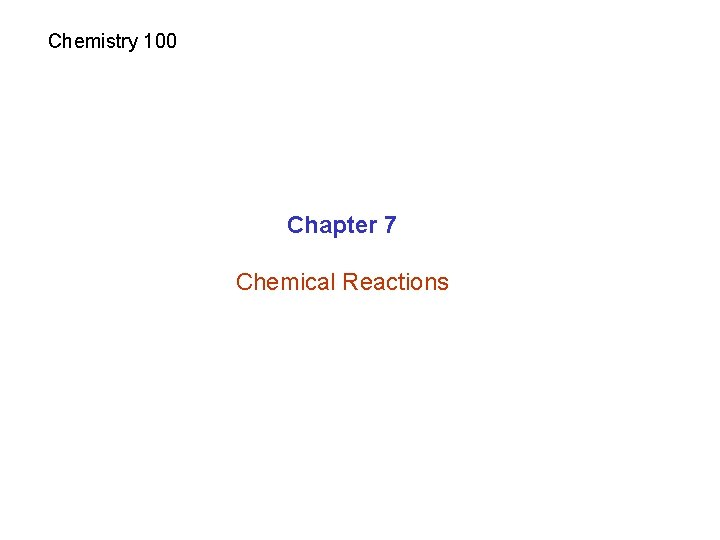Chemistry 100 Chapter 7 Chemical Reactions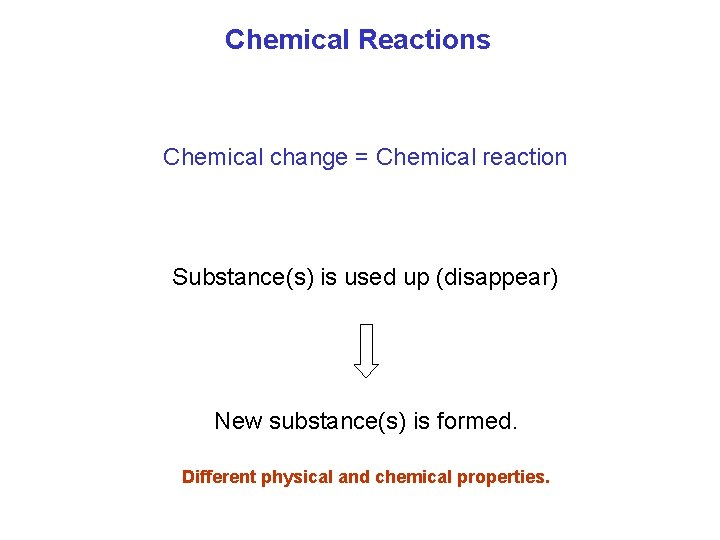Chemical Reactions Chemical change = Chemical reaction Substance(s) is used up (disappear) New substance(s) is formed. Different physical and chemical properties.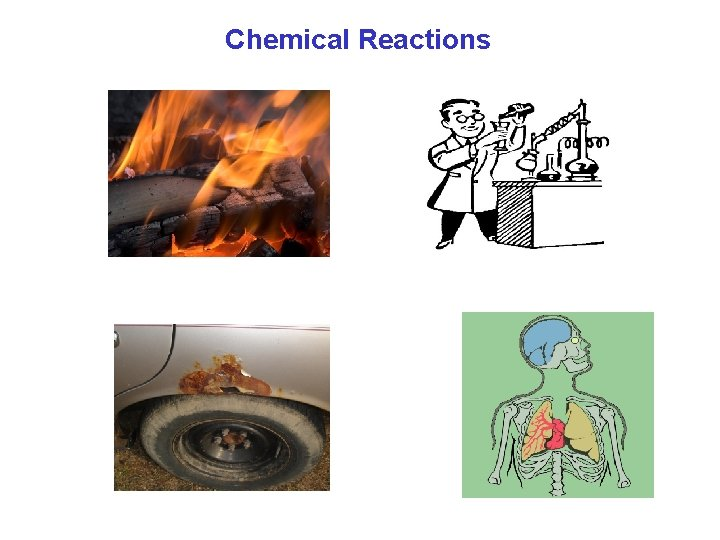Chemical ReactionsEvidence for chemical reactions 1. Color changes 2. A solid is formed (precipitation) 3. Bubbles form (gas) 4. Heat (and/or flame) is produced, or heat is absorbed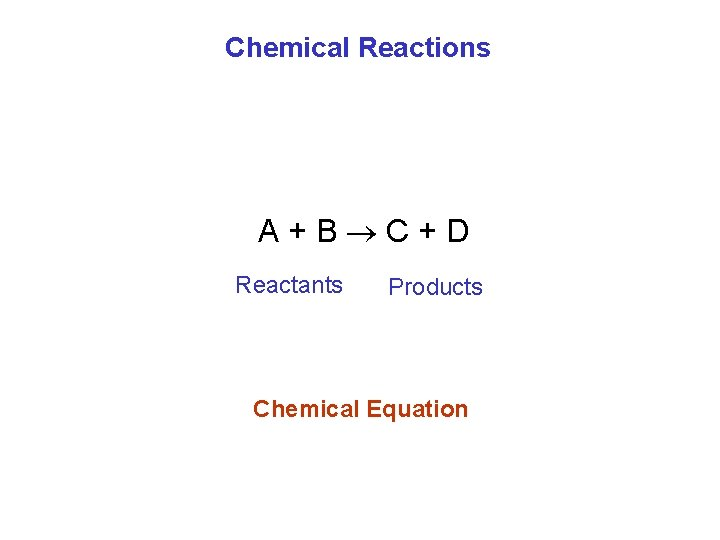Chemical Reactions A+B C+D Reactants Products Chemical Equation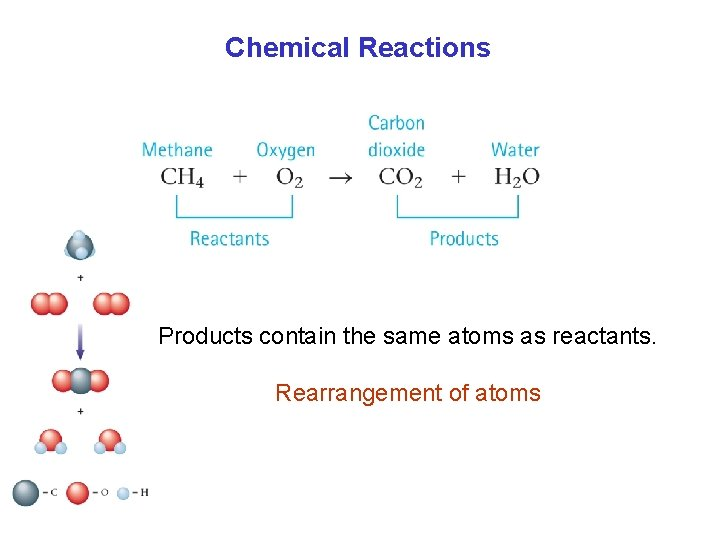Chemical Reactions Products contain the same atoms as reactants. Rearrangement of atoms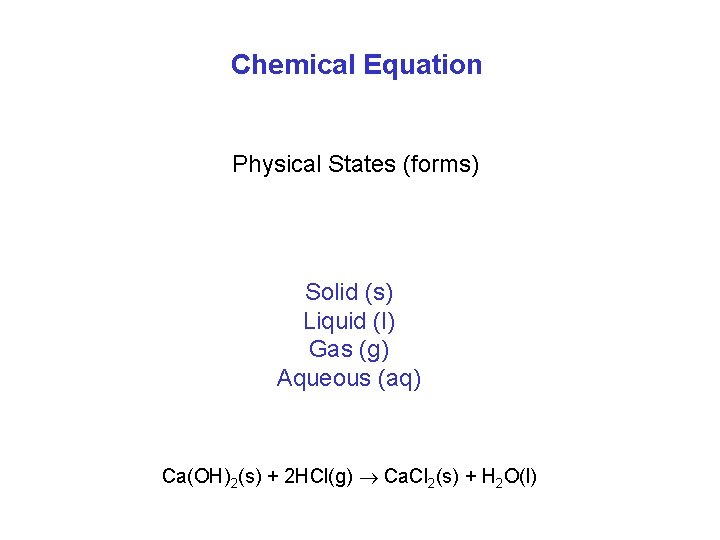Chemical Equation Physical States (forms) Solid (s) Liquid (l) Gas (g) Aqueous (aq) Ca(OH)2(s) + 2 HCl(g) Ca. Cl 2(s) + H 2 O(l)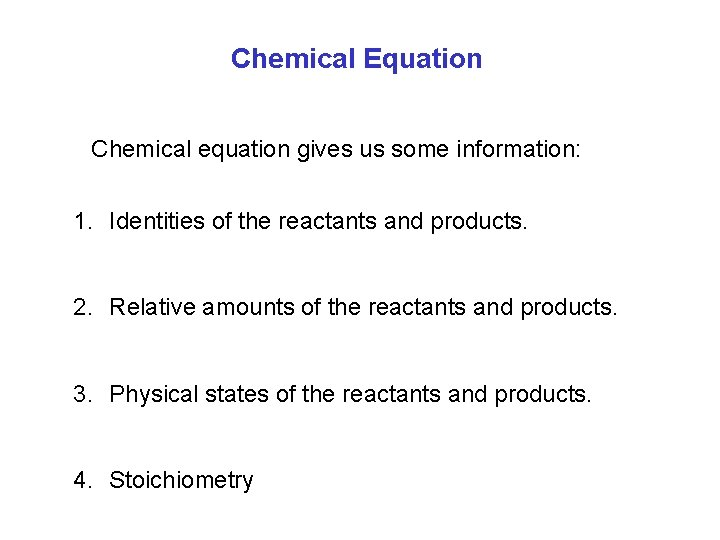Chemical Equation Chemical equation gives us some information: 1. Identities of the reactants and products. 2. Relative amounts of the reactants and products. 3. Physical states of the reactants and products. 4. StoichiometryType of chemical reactions 1. A + B AB Synthesis reaction (combination) 2 H 2 + O 2 2 H 2 O 2. AB A + B Decomposition (analysis) 2 Na. Cl 2 Na + Cl 2 3. A + BC AC + B Single replacement reaction Fe + Cu. SO 4 Fe. SO 4 + Cu 4. AB + CD AD + CB Double replacement reaction Na. Cl + Ag. NO 3 Na. NO 3 + Ag. Cl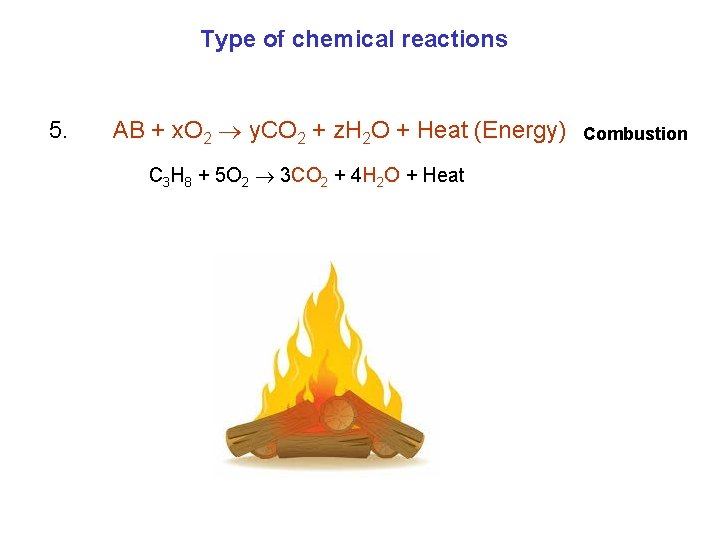Type of chemical reactions 5. AB + x. O 2 y. CO 2 + z. H 2 O + Heat (Energy) C 3 H 8 + 5 O 2 3 CO 2 + 4 H 2 O + Heat Combustion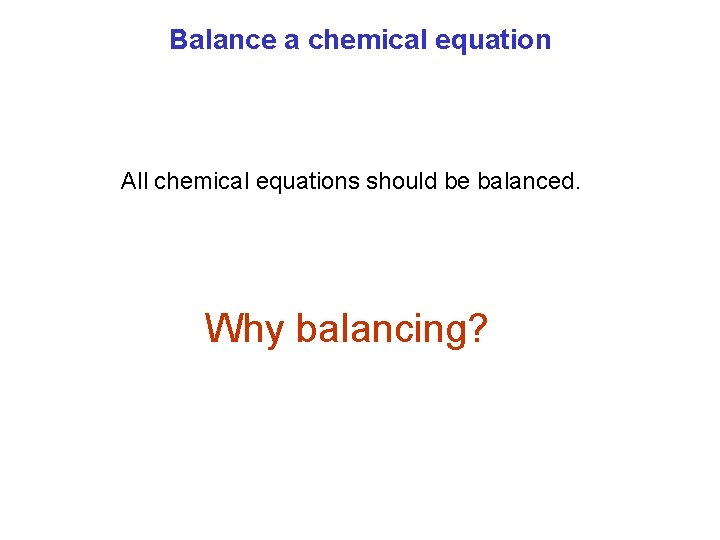Balance a chemical equation All chemical equations should be balanced. Why balancing?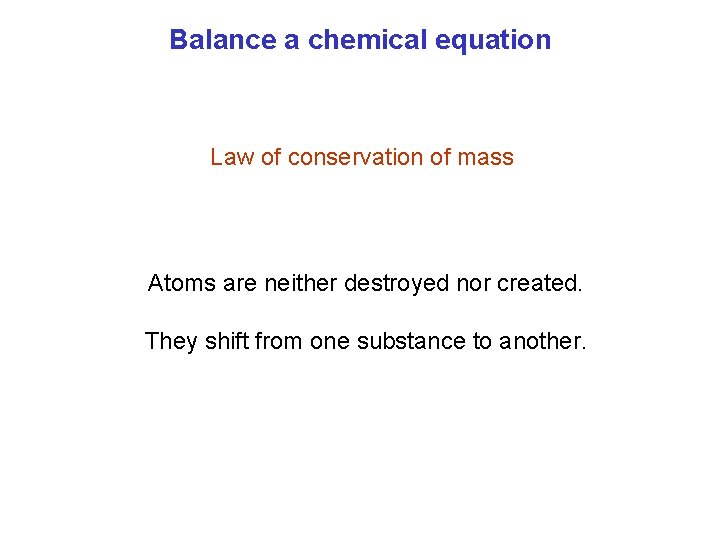Balance a chemical equation Law of conservation of mass Atoms are neither destroyed nor created. They shift from one substance to another.Balance a chemical equation 1. Begin with atoms that appear in only one compound on the left and right. 2. If an atom occurs as a free element, balance it last. 3. Change only coefficients (not formulas). C 3 H 8(g) + O 2(g) CO 2(g) + H 2 O(g) last Always double check!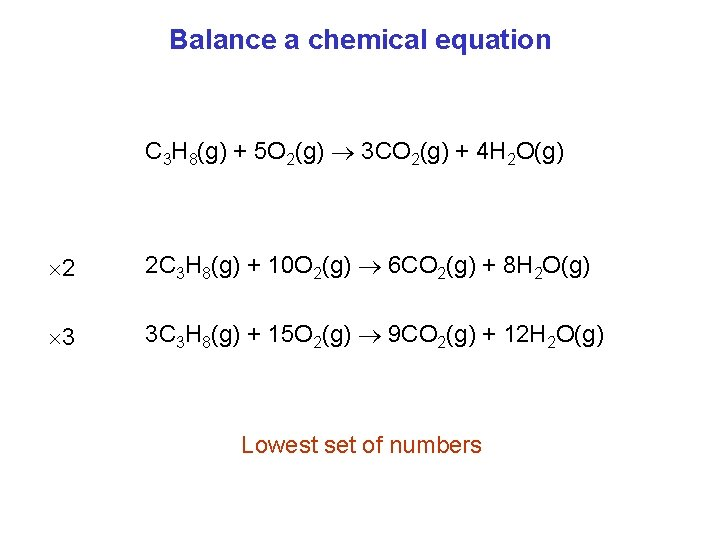Balance a chemical equation C 3 H 8(g) + 5 O 2(g) 3 CO 2(g) + 4 H 2 O(g) 2 2 C 3 H 8(g) + 10 O 2(g) 6 CO 2(g) + 8 H 2 O(g) 3 3 C 3 H 8(g) + 15 O 2(g) 9 CO 2(g) + 12 H 2 O(g) Lowest set of numbers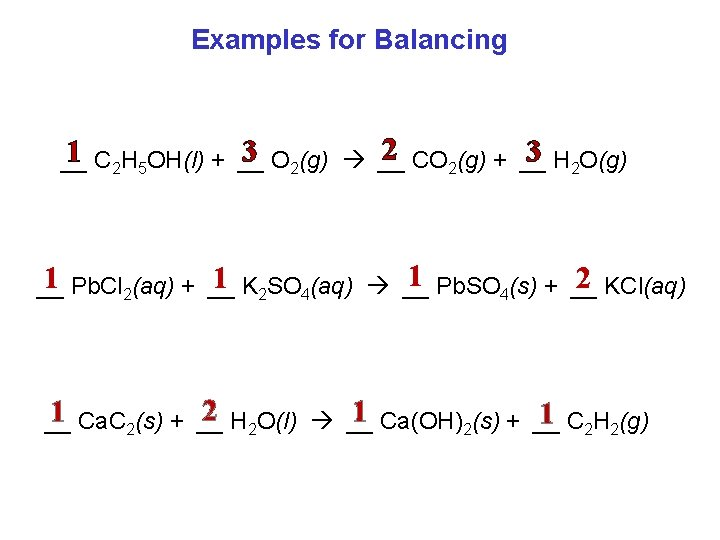Examples for Balancing 2 CO 2(g) + __ 1 C 2 H 5 OH(l) + __ 3 O 2(g) __ 3 H 2 O(g) __ 1 Pb. SO 4(s) + __ 2 KCl(aq) 1 Pb. Cl 2(aq) + __ 1 K 2 SO 4(aq) __ __ 2 H 2 O(l) __ 1 Ca. C 2(s) + __ 1 Ca(OH)2(s) + __ 1 C 2 H 2(g) __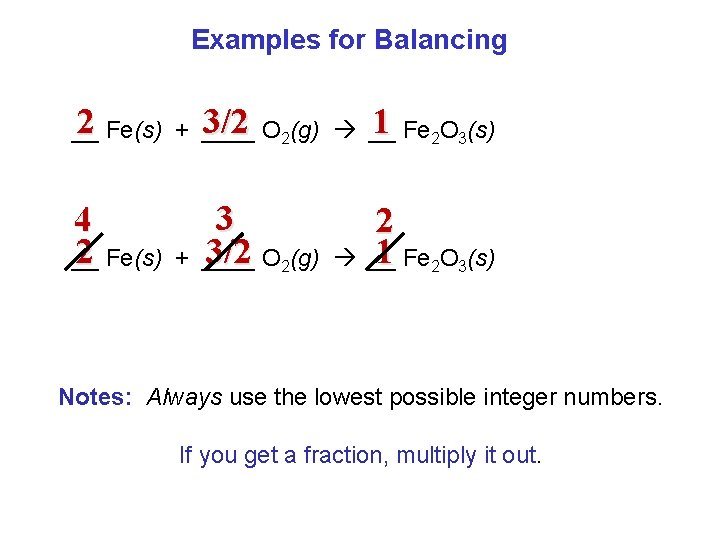Examples for Balancing 2 Fe(s) + ____ 3/2 O 2(g) __ 1 Fe 2 O 3(s) __ 4 2 Fe(s) __ + 3 3/2 O 2(g) ____ 2 1 Fe 2 O 3(s) __ Notes: Always use the lowest possible integer numbers. If you get a fraction, multiply it out.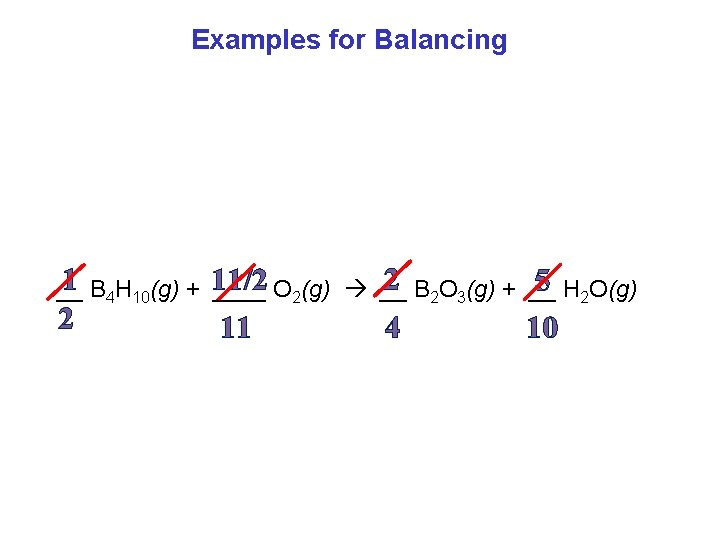Examples for Balancing 2 B 2 O 3(g) + __ 1 B 4 H 10(g) + 11/2 5 H 2 O(g) __ ____ O 2(g) __ 2 11 4 10Examples for Balancing • “Solid potassium reacts with water to form hydrogen gas and potassium hydroxide dissolved in solution. ” • Write and balance the chemical equation for this reaction. 1 K(s) + 2 H 2 O(l) → 1 KOH(aq) +1 H 2(g) 2 2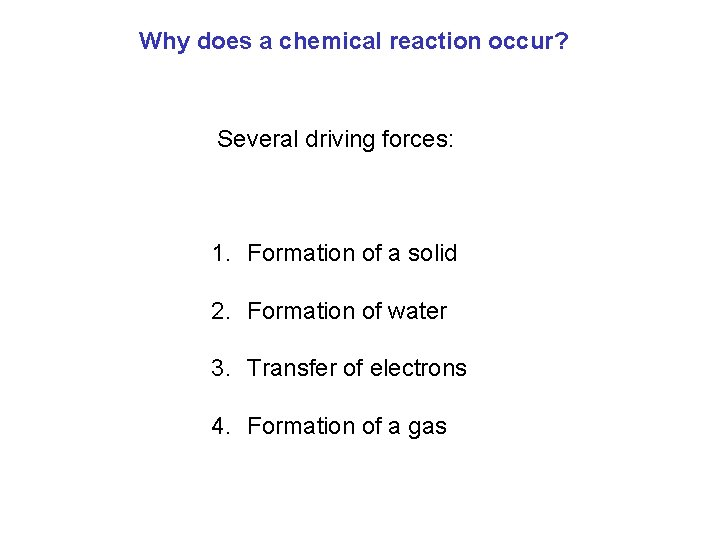Why does a chemical reaction occur? Several driving forces: 1. Formation of a solid 2. Formation of water 3. Transfer of electrons 4. Formation of a gasWhy does a chemical reaction occur? Several driving forces: 1. Formation of a solid 2. Formation of water 3. Transfer of electrons 4. Formation of a gasReactions in Aqueous Solutions Ionic compounds (Salt) Aqueous solution: solvent is waterReactions in Aqueous Solutions Chemical reactions that occur in water. 60% of our body is water. In our body reactions occur in the aqueous solution.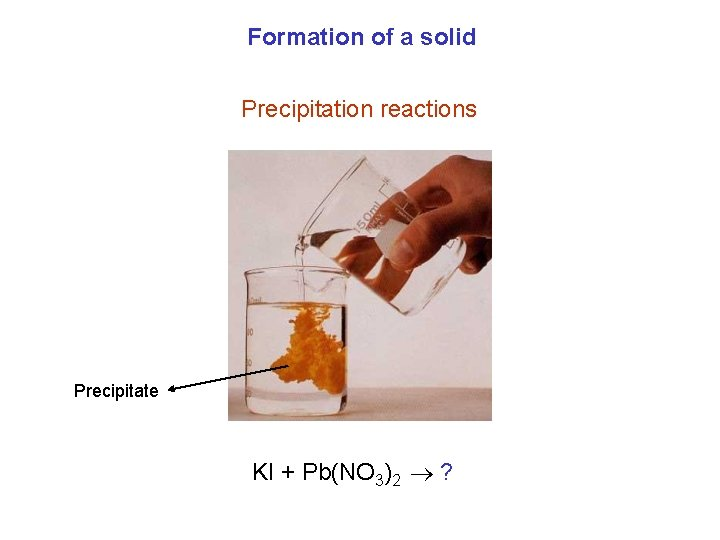Formation of a solid Precipitation reactions Precipitate KI + Pb(NO 3)2 ?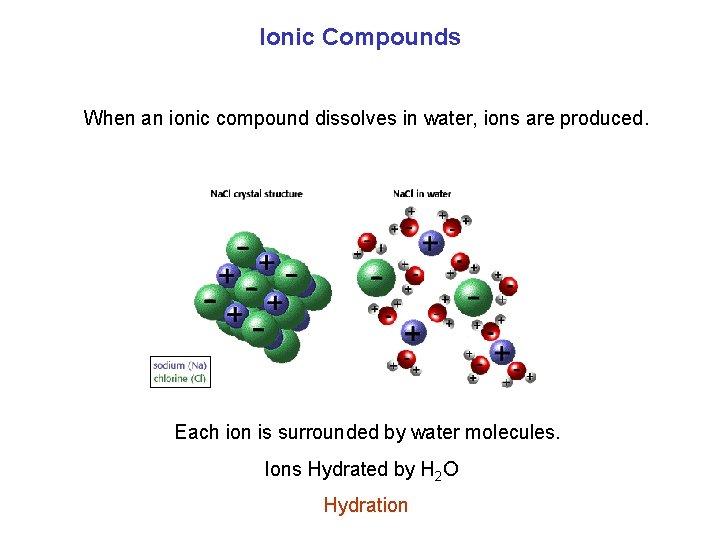Ionic Compounds When an ionic compound dissolves in water, ions are produced. Each ion is surrounded by water molecules. Ions Hydrated by H 2 O Hydration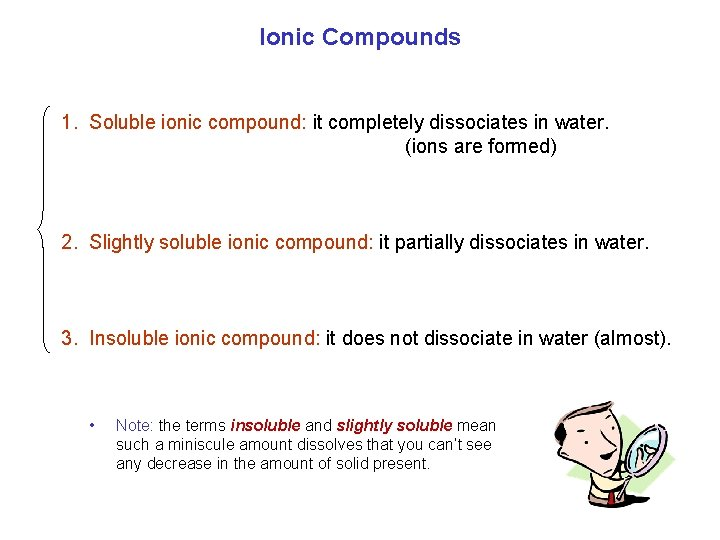Ionic Compounds 1. Soluble ionic compound: it completely dissociates in water. (ions are formed) 2. Slightly soluble ionic compound: it partially dissociates in water. 3. Insoluble ionic compound: it does not dissociate in water (almost). • Note: the terms insoluble and slightly soluble mean such a miniscule amount dissolves that you can’t see any decrease in the amount of solid present.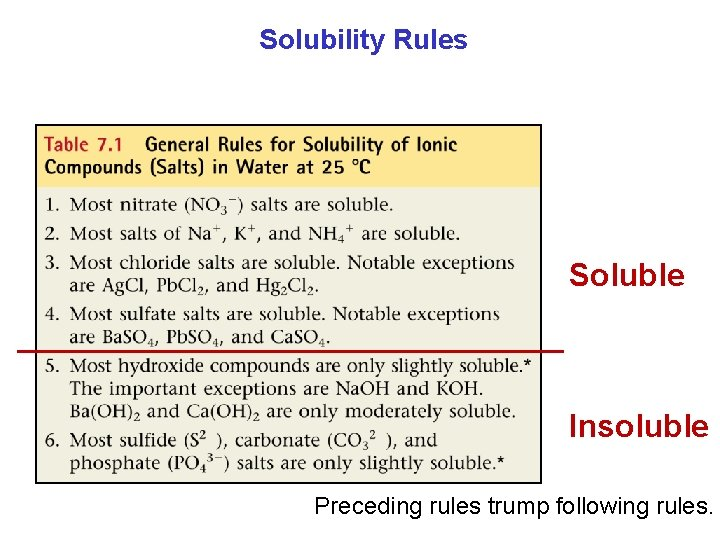Solubility Rules Soluble Insoluble Preceding rules trump following rules.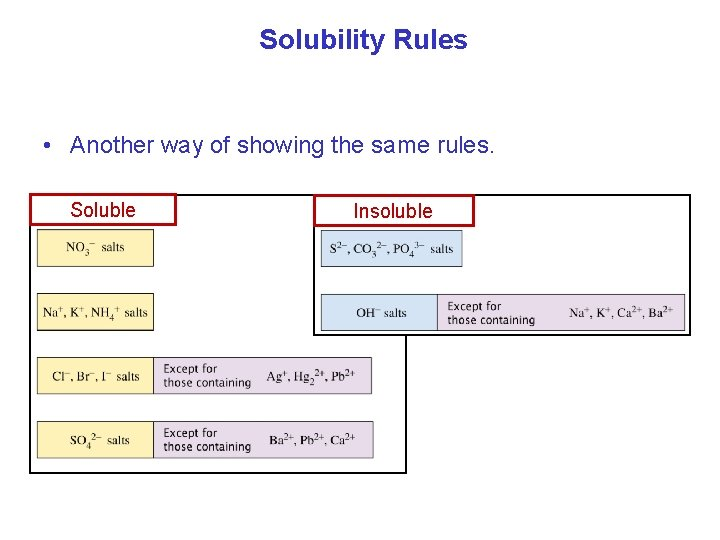Solubility Rules • Another way of showing the same rules. Soluble Insoluble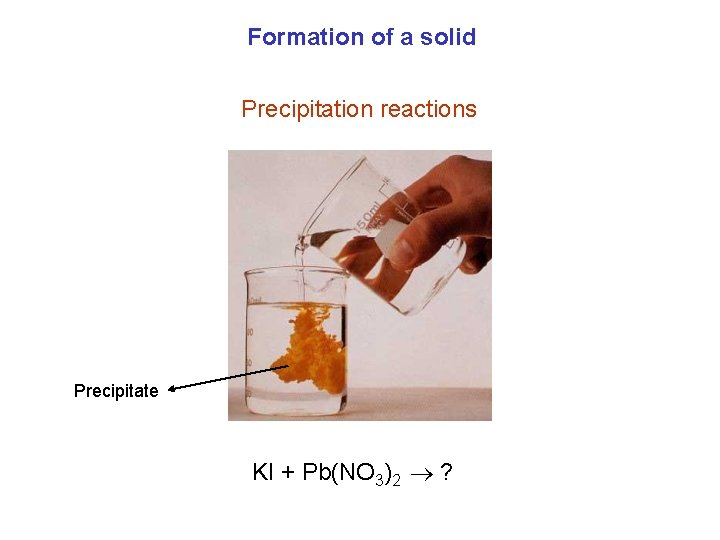Formation of a solid Precipitation reactions Precipitate KI + Pb(NO 3)2 ?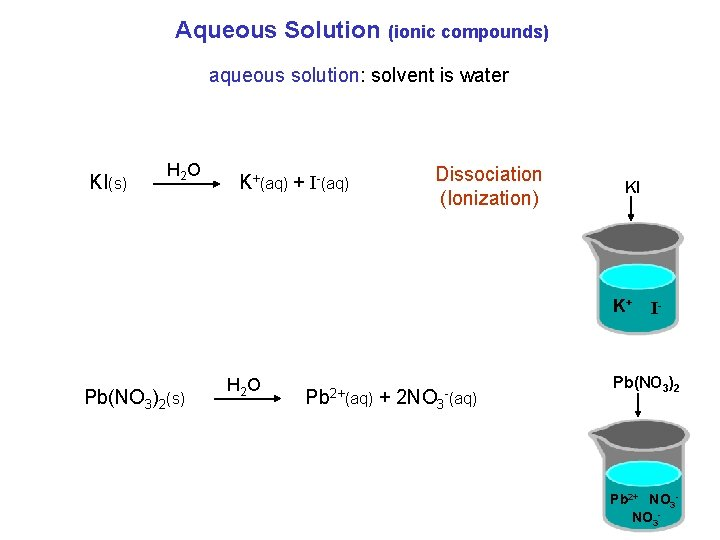Aqueous Solution (ionic compounds) aqueous solution: solvent is water KI(s) H 2 O K+(aq) + I-(aq) Dissociation (Ionization) KI K+ Pb(NO 3)2(s) H 2 O Pb 2+(aq) + 2 NO 3 -(aq) I- Pb(NO 3)2 Pb 2+ NO 3 -Aqueous Solution (ionic compounds) Sometimes the ions react with each other. Positive ions will interact with negative ions. KI Pb(NO 3)2 K+ I- Pb 2+ NO 3 - Sometimes ions stick together to form a solid (precipitate). 2 KI(aq) + Pb(NO 3)2(aq) Pb. I 2(s) + 2 KNO 3(aq)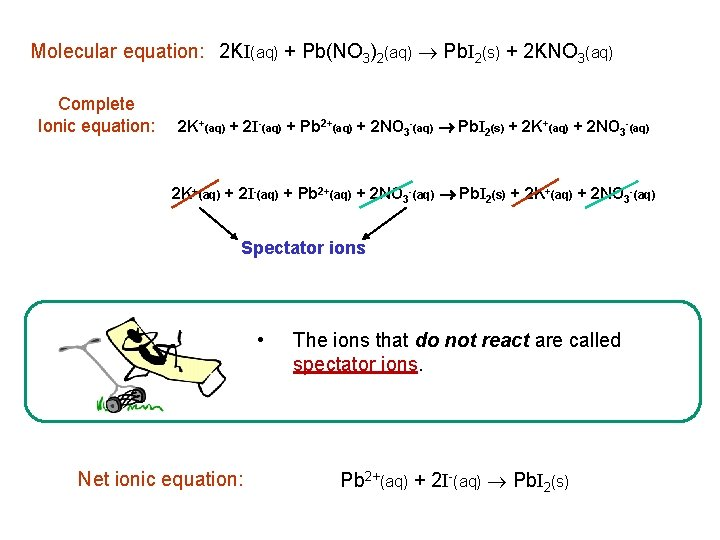Molecular equation: 2 KI(aq) + Pb(NO 3)2(aq) Pb. I 2(s) + 2 KNO 3(aq) Complete Ionic equation: 2 K+(aq) + 2 I-(aq) + Pb 2+(aq) + 2 NO 3 -(aq) Pb. I 2(s) + 2 K+(aq) + 2 NO 3 -(aq) Spectator ions • Net ionic equation: The ions that do not react are called spectator ions. Pb 2+(aq) + 2 I-(aq) Pb. I 2(s)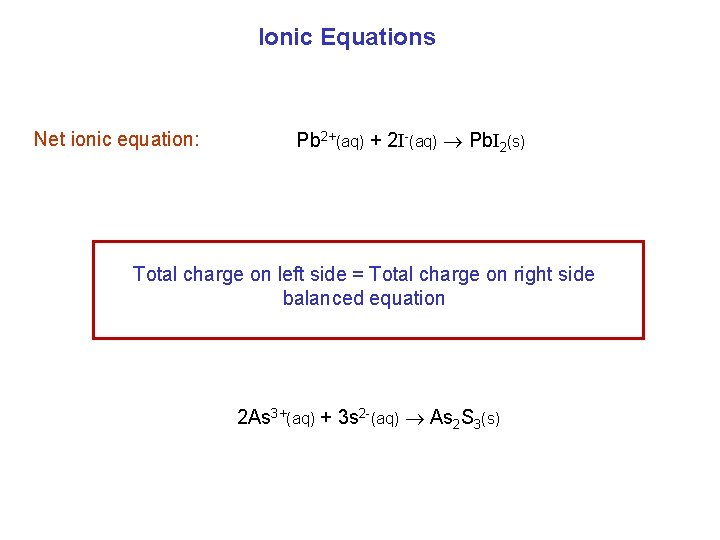Ionic Equations Net ionic equation: Pb 2+(aq) + 2 I-(aq) Pb. I 2(s) Total charge on left side = Total charge on right side balanced equation 2 As 3+(aq) + 3 s 2 -(aq) As 2 S 3(s)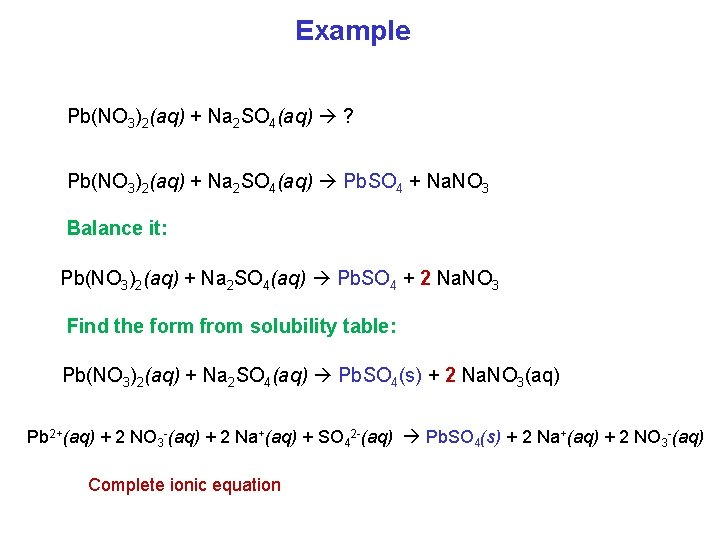Example Pb(NO 3)2(aq) + Na 2 SO 4(aq) ? Pb(NO 3)2(aq) + Na 2 SO 4(aq) Pb. SO 4 + Na. NO 3 Balance it: Pb(NO 3)2(aq) + Na 2 SO 4(aq) Pb. SO 4 + 2 Na. NO 3 Find the form from solubility table: Pb(NO 3)2(aq) + Na 2 SO 4(aq) Pb. SO 4(s) + 2 Na. NO 3(aq) Pb 2+(aq) + 2 NO 3 -(aq) + 2 Na+(aq) + SO 42 -(aq) Pb. SO 4(s) + 2 Na+(aq) + 2 NO 3 -(aq) Complete ionic equation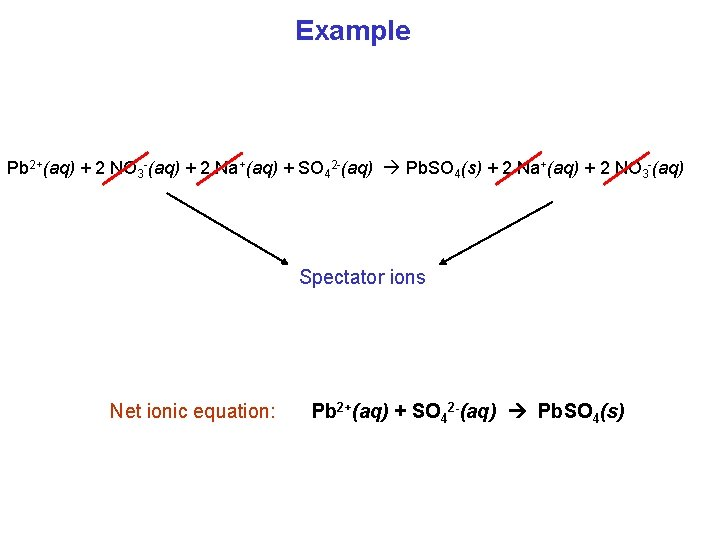Example Pb 2+(aq) + 2 NO 3 -(aq) + 2 Na+(aq) + SO 42 -(aq) Pb. SO 4(s) + 2 Na+(aq) + 2 NO 3 -(aq) Spectator ions Net ionic equation: Pb 2+(aq) + SO 42 -(aq) Pb. SO 4(s)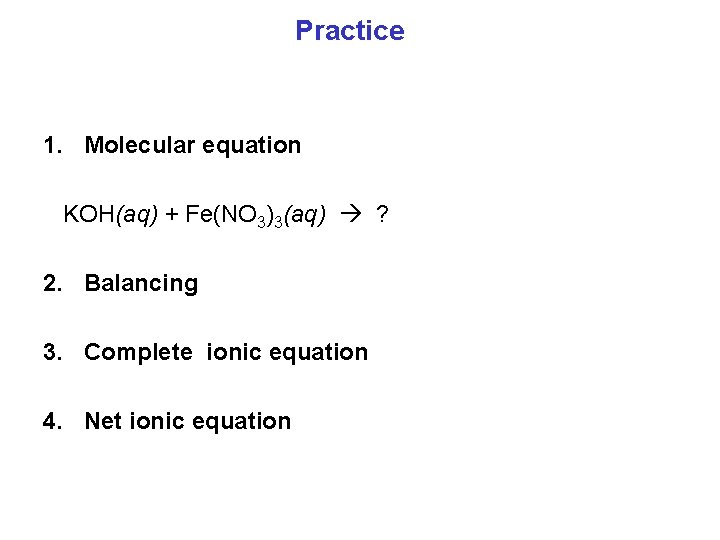Practice 1. Molecular equation KOH(aq) + Fe(NO 3)3(aq) ? 2. Balancing 3. Complete ionic equation 4. Net ionic equation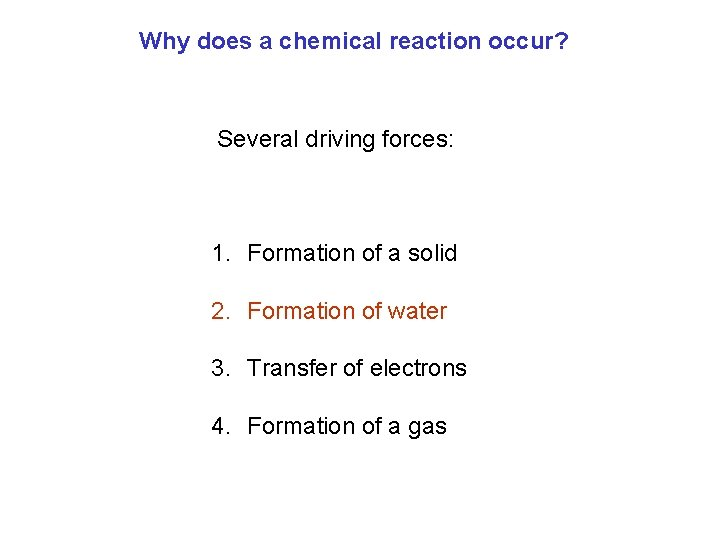Why does a chemical reaction occur? Several driving forces: 1. Formation of a solid 2. Formation of water 3. Transfer of electrons 4. Formation of a gasAcids and Bases Acids: sour Bases: bitter or saltyAcid-Base Reactions Neutralization Strong acid + Strong base → Salt + H 2 O HCl(aq) + Na. OH(aq) H+(aq) + Cl-(aq) + Na+(aq) + OH-(aq) H+(aq) + OH-(aq) Na. Cl(aq) + H 2 O(l) Na+(aq) + Cl-(aq) + H 2 O(l) The only chemical change is the formation of water.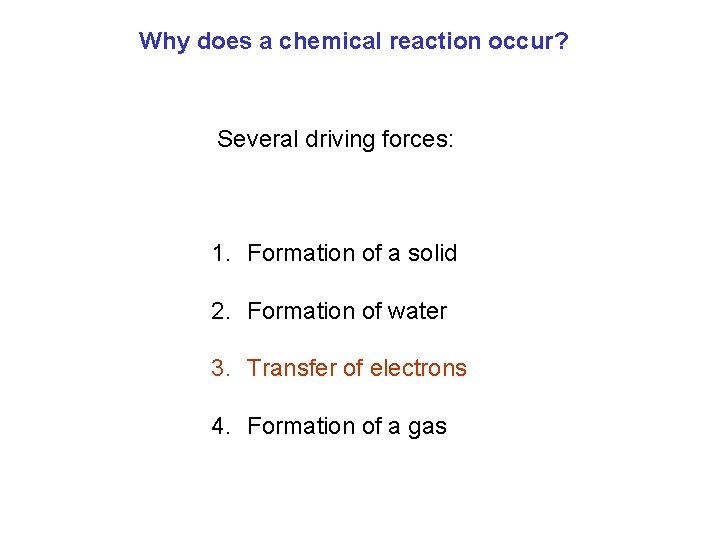Why does a chemical reaction occur? Several driving forces: 1. Formation of a solid 2. Formation of water 3. Transfer of electrons 4. Formation of a gas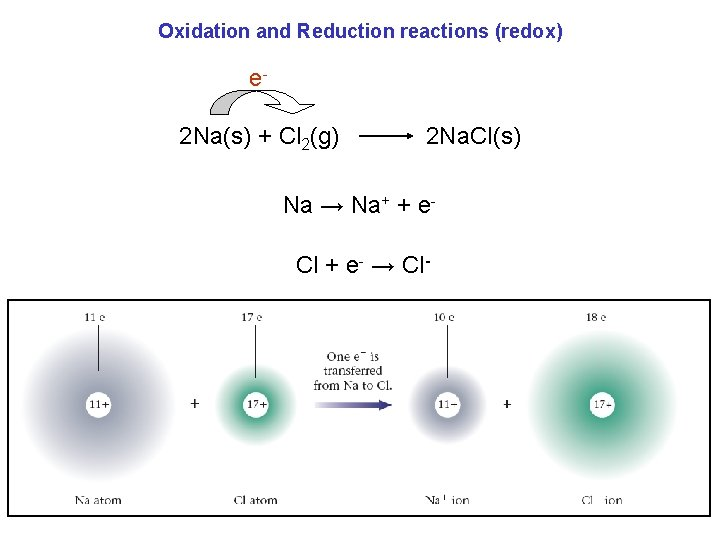Oxidation and Reduction reactions (redox) e 2 Na(s) + Cl 2(g) 2 Na. Cl(s) Na → Na+ + e. Cl + e- → Cl-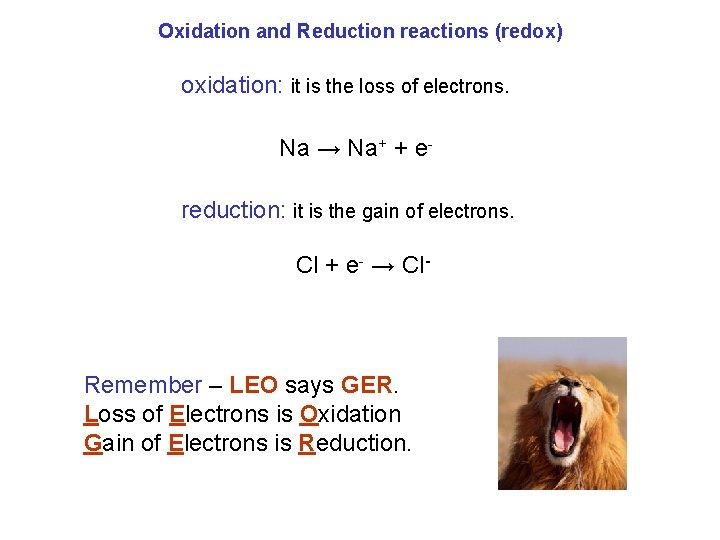Oxidation and Reduction reactions (redox) oxidation: it is the loss of electrons. Na → Na+ + ereduction: it is the gain of electrons. Cl + e- → Cl- Remember – LEO says GER. Loss of Electrons is Oxidation Gain of Electrons is Reduction.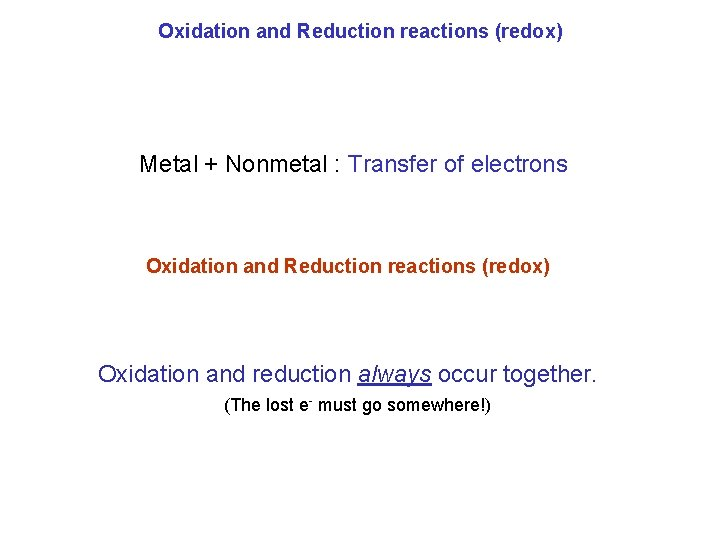Oxidation and Reduction reactions (redox) Metal + Nonmetal : Transfer of electrons Oxidation and Reduction reactions (redox) Oxidation and reduction always occur together. (The lost e- must go somewhere!)Oxidation and Reduction reactions (redox) oxidation: it is the loss of electrons. reduction: it is the gain of electrons. Zn(s) + Cu 2+(aq) Zn 2+(aq) + Cu(s) redox reaction Zn(s) Zn 2+(aq) + 2 e- Zn is oxidized (reducing agent) Cu 2+(aq) + 2 e- Cu(s) Cu 2+ is reduced (oxidizing agent)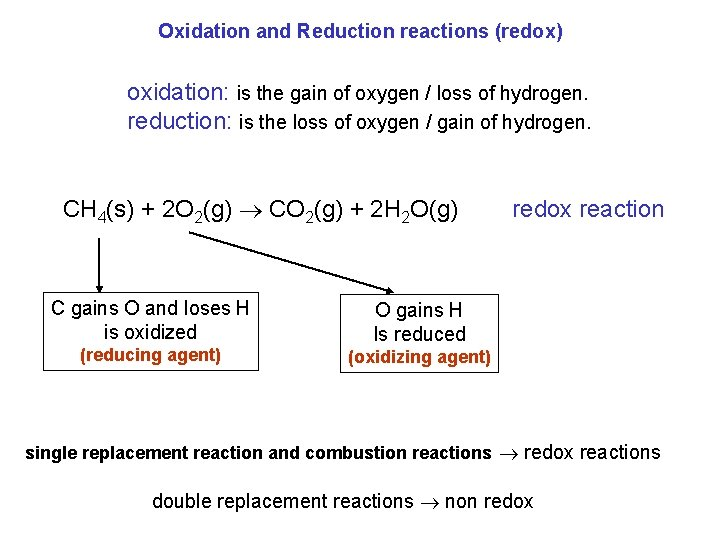Oxidation and Reduction reactions (redox) oxidation: is the gain of oxygen / loss of hydrogen. reduction: is the loss of oxygen / gain of hydrogen. CH 4(s) + 2 O 2(g) CO 2(g) + 2 H 2 O(g) C gains O and loses H is oxidized (reducing agent) redox reaction O gains H Is reduced (oxidizing agent) single replacement reaction and combustion reactions redox reactions double replacement reactions non redox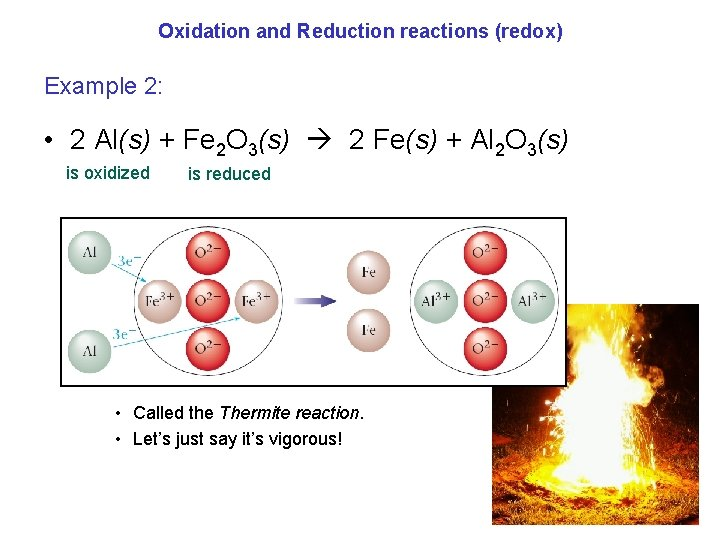Oxidation and Reduction reactions (redox) Example 2: • 2 Al(s) + Fe 2 O 3(s) 2 Fe(s) + Al 2 O 3(s) is oxidized is reduced • Called the Thermite reaction. • Let’s just say it’s vigorous!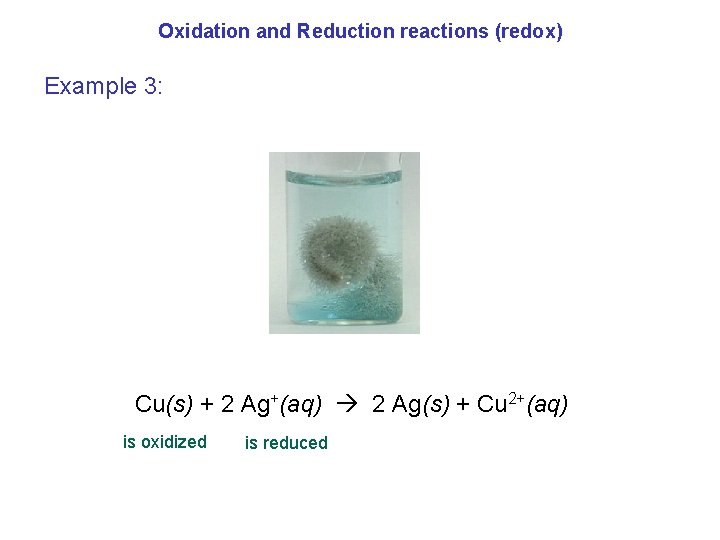Oxidation and Reduction reactions (redox) Example 3: Cu(s) + 2 Ag+(aq) 2 Ag(s) + Cu 2+(aq) is oxidized is reduced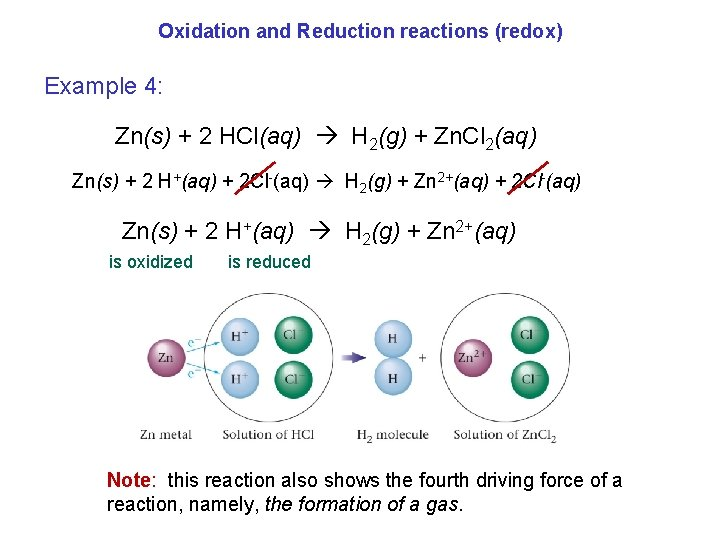Oxidation and Reduction reactions (redox) Example 4: Zn(s) + 2 HCl(aq) H 2(g) + Zn. Cl 2(aq) Zn(s) + 2 H+(aq) + 2 Cl-(aq) H 2(g) + Zn 2+(aq) + 2 Cl-(aq) Zn(s) + 2 H+(aq) H 2(g) + Zn 2+(aq) is oxidized is reduced Note: this reaction also shows the fourth driving force of a reaction, namely, the formation of a gas.Oxidation and Reduction reactions (redox) Example 5: 2 Mg(s) + O 2(g) 2 Mg. O(s) is oxidized is reduced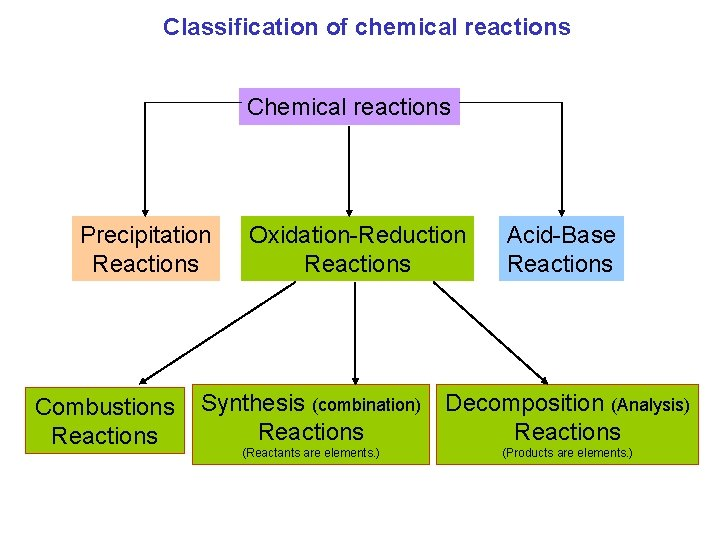Classification of chemical reactions Chemical reactions Precipitation Reactions Combustions Reactions Oxidation-Reduction Reactions Acid-Base Reactions Synthesis (combination) Reactions Decomposition (Analysis) Reactions (Reactants are elements. ) (Products are elements. )